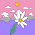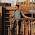### Efficiency of a Transformer

Efficiency of a Transformer
Due to the losses in a transformer, the output power of a transformer is less than the input power supplied.
...           Power output = Power input - Total losses
...           Power input = Power output + Total losses
= Power output + Pi  + Pcu
The efficiency of any device is defined as the ratio of the power output to power input. So for a transformer the efficiency can be expresses as,
η = Power output/power input
...                 η = Power output/(power output + Pi  + Pcu )
Now power output = V2 I2 cos Φ
where          cos Φ = Load power factor
The transformer supplies full load of current I2 and with terminal voltage V2.
Pcu  = Copper losses on full load = I22 R2e
...               η = (V2 I2 cos Φ2  )/(V2 I2 cos Φ2  + Pi  + I22 R2e)
But            V2 I2 = VA rating of a transformer
...               η  = (VA rating x cos Φ) / (VA rating x cos Φ + Pi  + I22 R2e)
This is full load percentage efficiency with,
I2 = Full load secondary current
But if the transformer is subjected to fractional load then using the appropriate values of various quantities, the efficiency can be obtained.
Let n =Fraction by which load is less than full load = Actual load/Full load
For example, if transformer is subjected to half load then,
n = Half load/Full load = (1/2)/2 = 0.5
when load changes, the load current changes by same proportion.
...     new I2 = n (I2) F.L.
Similarly the output V2 I2 cosΦ2  also reduces by the same fraction. Thus fraction of VA rating is available at the output.
Similarly as copper losses are proportional to square of current then,
new Pcu  = n2 (Pcu  ) F.L.
Key Point : So copper losses get reduced by n2.
In general for fractional load the efficiency is given by,

where n = Fraction by which load power factor lagging, leading and unity the efficiency expression does not change, and remains same.
Example : A 4 KVA, 200/400 V, 50 Hz, single phase transformer has equivalent resistance referred to primary as 0.15 Ω Calculate,
i) The total copper losses on full load.
ii) The efficiency while supplying full load at 0.9 p.f. lagging.
iii) The efficiency while supplying half load at 0.8 p.f. leading.
Assume total iron losses equal to 60 W.

Solution : The given values are,
V1 = 200 V, V2 = 400 V, S = 4 KVA, R1e= 0.15 Ω , P= 60 W
K = 400/200 = 2
...           R2e = K2 R1e = (2)2 x 0.15 = 0.6 Ω
(I2)F.L. = KVA/V2 = 4 x 103/400 = 10 A
(i) Total copper losses on full load,
(Pcu)F.L. = {(I2) F.L.}2 R2e = (10)2 x 0.6 = 60 W
(ii) cos Φ = 0.9 lagging and full load

(iii) cos Φ = 0.8 leading, half load
As half load,           n = 0.5
Sponsored Articles :

#### 2 comments:

1.Thanks a lot!

2.Is that Pi = 60 W is given in the question?